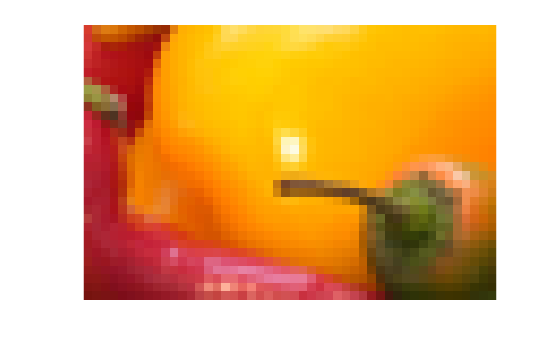Read tile data using RGBA interface

## Syntax

``[RGB,alpha] = readRGBATile(t,row,col)``

## Description

example

````[RGB,alpha] = readRGBATile(t,row,col)` uses the RGBA interface to read a tile from the TIFF file associated with the `Tiff` object `t`. The tile is specified by `row` and `col` which are the one-based row and column numbers of any pixel in the requested tile.The `readRGBATile` function trims the area in a tile that falls outside of the `ImageLength` and `ImageWidth` boundaries. Therefore, image data from tiles that occur on the bottom edge or the right edge of the image can have different dimensions.```

## Examples

collapse all

Read a tile of data from a TIFF image file using the RGBA interface.

Create a `Tiff` object for the image file.

`t = Tiff('peppers_RGB_tiled.tif','r');`

Get the number of tiles and tile size.

`numTiles = numberOfTiles(t)`
```numTiles = 36 ```
`tileSize = [getTag(t,'TileLength') getTag(t,'TileWidth')]`
```tileSize = 1×2 32 48 ```

Read the tile containing the `100`th row and `100`th column from the image. The `readRGBATile` function trims the area in a tile that is outside of the `ImageLength` and `ImageWidth` boundaries. Therefore, image data from tiles that occur on the right edge or the bottom edge of the image can have different dimensions.

```row = 100; col = 100; [RGB,A] = readRGBATile(t,row,col);```

Display the image.

`imshow(RGB,'InitialMagnification','fit') % magnify for display`Close the `Tiff` object.

`close(t);`

## Input Arguments

collapse all

`Tiff` object representing a TIFF file. Use the `Tiff` function to create the object.

Row number, specified as positive integer. Specify `row` as a one-based row index of any pixel in the tile.

Example: `100`

Data Types: `double`

Column number, specified as positive integer. Specify `col` as a one-based column index of any pixel in the tile.

Example: `57`

Data Types: `double`

## Output Arguments

collapse all

Image tile data, returned as an `m`-by-`n`-by-`3` numeric array. Where `m` and `n` are the height and width of the tile, respectively.

The value in the `TileLength` tag determines the number of rows and the `TileWidth` tag determines the number of columns in the tile data.

The `readRGBATile` function can transform the pixel values based on specifications in these tags:

 `PhotometricInterpretation` `BitsPerSample` `SamplesPerPixel` `Orientation` `ExtraSamples` `ColorMap`

Alpha matting associated with the image tile, returned as a numeric array. The number of rows and columns in the alpha matting data are the same as the tile data.

If the image does not have associated alpha matting, then `alpha` is a matrix with all values set to `255` (transparent).

## Algorithms

collapse all

### References

This function corresponds to the `TIFFReadRGBATile` function in the LibTIFF C API. To use this function, you must be familiar with the TIFF specification and technical notes. View this documentation at `LibTIFF - TIFF Library and Utilities`.